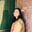Trusted answers to developer questions
Trusted Answers to Developer Questions

Related Tags

c

# What is log1pl() in C?Umme Ammara

Grokking Modern System Design Interview for Engineers & Managers

Ace your System Design Interview and take your career to the next level. Learn to handle the design of applications like Netflix, Quora, Facebook, Uber, and many more in a 45-min interview. Learn the RESHADED framework for architecting web-scale applications by determining requirements, constraints, and assumptions before diving into a step-by-step design process.The log1pl function is a C library function that returns the natural logarithm of the sum of one and the argument. For example, if the argument provided is x, the function calculates the natural logarithm of (1 + x), as shown below:

To use the log1pl function, we need to include the math.h header file, as shown below:

#include <math.h>


## Syntax

The log1pl function takes a single argument and returns a single value. It is declared as follows:

## Parameter

The log1pl function takes a single argument of the long double type.

## Return Value

The log1pl function returns a single value of the long double type:

• If x is less than -1, a domain error occurs

• If x is equal to -1, a range error may occur

• If x is equal to 0, the return value will be 0

Hence, arguments with values greater than -1 will produce correct results, i.e., the natural logarithm (1+x).

## Example

The code below shows the use of the log1pl function in C:

#include <stdio.h>#include <math.h>int main() {  //Declare x   long double x  = 0.8889;   //Call the function   long double ans = log1pl (x);  //Display result   printf ("The natural log of (x+1) is: %Lf\n", ans);    return 0;}

RELATED TAGS

c

CONTRIBUTORUmme Ammara